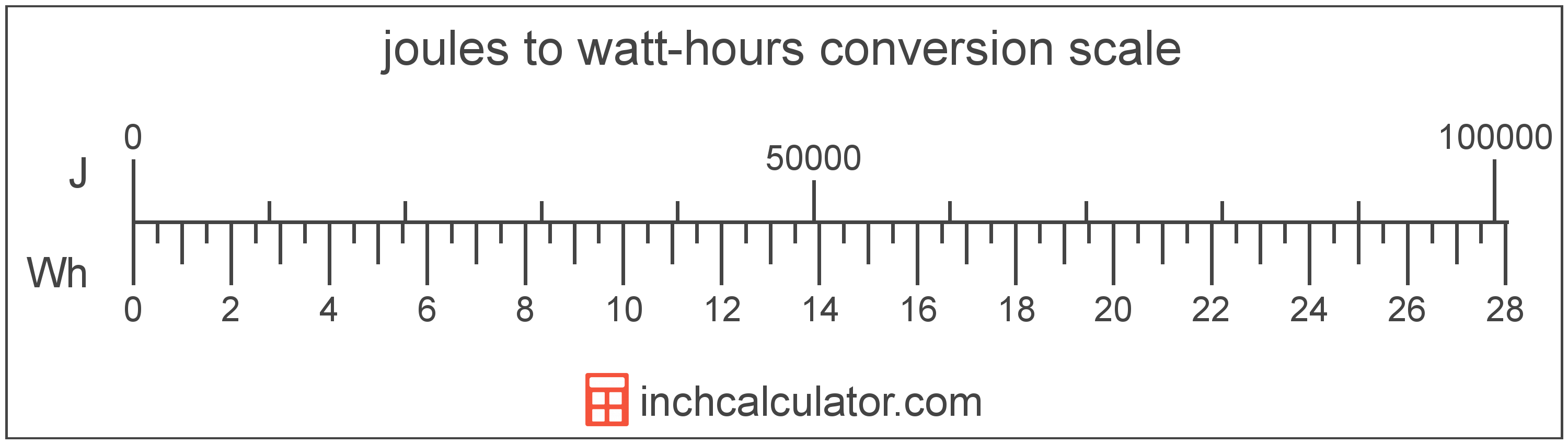# Watt-Hours to Joules Converter

Enter the energy in watt-hours below to get the value converted to joules.

Results in Joules:1 Wh = 3,600 J

Do you want to convert joules to watt-hours?

## How to Convert Watt-Hours to Joules

To convert a measurement in watt-hours to a measurement in joules, multiply the energy by the following conversion ratio: 3,600 joules/watt-hour.

Since one watt-hour is equal to 3,600 joules, you can use this simple formula to convert:

joules = watt-hours × 3,600

The energy in joules is equal to the energy in watt-hours multiplied by 3,600.

For example, here's how to convert 5 watt-hours to joules using the formula above.
joules = (5 Wh × 3,600) = 18,000 J### How Many Joules Are in a Watt-Hour?

There are 3,600 joules in a watt-hour, which is why we use this value in the formula above.

1 Wh = 3,600 J

## What is a Watt-Hour?

The watt-hour is a measure of electrical energy equal to one watt of power over a one hour period.

Watt-hours are usually abbreviated as Wh, although the formally adopted expression is W·h. The abbreviation W h is also sometimes used. For example, 1 watt-hour can be written as 1 Wh, 1 W·h, or 1 W h.

In formal expressions, the centered dot (·) or space is used to separate units used to indicate multiplication in an expression and to avoid conflicting prefixes being misinterpreted as a unit symbol.

## What is a Joule?

The joule is the energy equal to the force on an object of one newton at a distance of one meter. One joule is equal to the heat energy dissipated by the current of one ampere through one ohm of resistance for one second.

One joule is also equal to the energy needed to move an electric charge of one coulomb through a potential difference of one volt. In addition, one joule is also equal to the one watt-second.

The joule is the SI derived unit for energy in the metric system. Joules can be abbreviated as J; for example, 1 joule can be written as 1 J.

## Watt-Hour to Joule Conversion Table

Table showing various watt-hour measurements converted to joules.
Watt-hours Joules
0.001 Wh 3.6 J
0.002 Wh 7.2 J
0.003 Wh 10.8 J
0.004 Wh 14.4 J
0.005 Wh 18 J
0.006 Wh 21.6 J
0.007 Wh 25.2 J
0.008 Wh 28.8 J
0.009 Wh 32.4 J
0.01 Wh 36 J
0.02 Wh 72 J
0.03 Wh 108 J
0.04 Wh 144 J
0.05 Wh 180 J
0.06 Wh 216 J
0.07 Wh 252 J
0.08 Wh 288 J
0.09 Wh 324 J
0.1 Wh 360 J
0.2 Wh 720 J
0.3 Wh 1,080 J
0.4 Wh 1,440 J
0.5 Wh 1,800 J
0.6 Wh 2,160 J
0.7 Wh 2,520 J
0.8 Wh 2,880 J
0.9 Wh 3,240 J
1 Wh 3,600 J

## References

1. Bureau International des Poids et Mesures, The International System of Units (SI), 9th edition, 2019, https://www.bipm.org/documents/20126/41483022/SI-Brochure-9-EN.pdf
2. International Bureau of Weights and Measures, The International System of Units, 9th Edition, 2019, https://www.bipm.org/documents/20126/41483022/SI-Brochure-9-EN.pdf